Journal DetailsFormat
Journal
eISSN
2083-134X
First Published
16 Apr 2011
Publication timeframe
4 times per year
Languages
EnglishOpen Access

# Structural, optical and dielectric studies of lithium sulphate monohydrate single crystals

###### Accepted: 15 Nov 2016
Journal DetailsFormat
Journal
eISSN
2083-134X
First Published
16 Apr 2011
Publication timeframe
4 times per year
Languages
English

Optical, dielectric, and thermal properties of lithium sulphate monohydrate crystals grown by slow evaporation method have been studied. The crystal structure was resolved by direct methods using single crystal X-ray diffraction data collected at room temperature and refined by full-matrix least-squares procedures to a final R-value of 0.0174. Plasma energy, Penn gap, Fermi energy and electronic polarizability of the grown crystal were calculated from single crystal XRD data. The electronic polarizability of lithium sulfate monohydrate was also calculated and compared with the theoretical data using Clausius-Mossotti equation. Optical band gap calculated from optical data for the grown crystal is 4.49 eV. Fourier Transform Infrared Spectroscopy study confirmed the presence of water in the crystal structure. The AC conductivity, dielectric constant and dielectric loss of the grown crystal were systemically investigated, showing a peak at about 130 °C which could be attributed to the water molecules in the crystal structure. The anomalous dielectric properties shown by the crystal have been correlated with its thermal behavior. The title crystal obeys Jonscher’s power law relation; σ(ω) = σo+ Aωs, with temperature dependent exponent s < 1. The activation energy calculated for the material is 0.24 eV and suggests protonic conduction by hopping mechanism in addition to cationic conduction by lithium ions. The micro-indentation study was also carried out which revealed that the crystal belongs to a category of soft materials.

#### Keywords

Introduction

Materials possessing pyroelectric, piezoelectric and nonliear optical properties have been given immense interest over recent years. Nonlinear optical (NLO) materials play an important role in optical communication, laser technology, frequency conversion and data storage technology . Zeiler  first determined the crystal structure of lithium sulphate monohydrate (LSMH) without localization of hydrogen atoms, which was later confirmed by Larson et al. . The structural knowledge of LSMH was completed by Ozerov et al. , after adding the hydrogen positions determined from a neutron diffraction study. LSMH crystals show the properties of pyroelectricity and piezoelectricity [10, 11]. The various studies carried out on the title compound include non-linear optical behavior, Raman spectroscopy, neutron diffraction at different temperatures and determination of pyroelectric coefficient . The growth by slow evaporation of LSMH crystals has been studied by Priya et al. . The nonlinear optical polarization, temperature dependences of the pyroelectric coefficient and of the spontaneous polarization of LSMH have also been investigated .

Recently, the effect of dopants such as urea, thiourea and copper on the optical and electrical properties of lithium sulphate crystals has been investigated by Mageshwari et al. . LSMH crystals grown by slow evaporation and Sankaranaryanan-Ramasamy method show negative solubility behavior as reported by Boopathi et al. .

Crystals with hydrogen-bonding and lithium based alkali cations are well known for their high protonic conductivity which increases significantly in their high temperature super-ionic phases . These systems have high cationic mobilities, and hence, exhibit good fast-ion conductivity at higher temperatures [25, 26]. Such kind of materials finds application in solid state batteries and fuel cells where they can be used as electrolyte materials or fast-ionic conductors. Proton conductors also find application for the development of new generation of energy storage and fuel cells. Lithium sulphate is an excellent lithium ion conductor in the high temperature α-phase but it is also a proton conductor in hydrogen-containing atmospheres . Crystalline materials in which the structural arrangement allows the motion of alkali ions along specific crystallographic axes has been a prime focus of investigations.

In the present work, the anomalous dielectric behavior of LSMH crystals has been reported. It was observed that AC conductivity of the present crystal system is controlled by hopping mechanism. The growth aspect and the characterization studies, such as SXRD, UV-Vis, FT-IR, TGA/DTG, and microhardness of LSMH single crystals have been presented. Some numerical calculations were also done for determination of polarizability using Clausius-Mossotti equation and Penn analysis.

Materials and methods

Lithium sulphate monohydrate (AR grade) was dissolved in double distilled water and stirred continuously for more than 6 hours to prepare a saturated solution. The prepared solution was filtered and kept in a beaker closed with perforated covers to ensure dust free atmosphere. The solution was kept for slow evaporation at room temperature. To increase the purity of synthesized crystals, they were subjected to recrystallization process and the crystals were harvested after attaining the optimal shape and size (8 mm × 4 mm × 1 mm) after 4 weeks. Bruker AXS Kappa APEX II single crystal X-ray diffractometer was used to obtain the data for determination of LSMH crystal structure. The structure was then resolved by direct methods and refined by full-matrix least squares cycles in SHELXL-97. The UV-Vis spectra of the grown crystals were obtained by using a spectrophotometer (UV-VIS-NIR Lambda 950S PerkinElmer) in the wavelength range of 190 nm to 900 nm. The FT-IR spectrum of the crystal in the wave number range of 400 cm−1 to 4000 cm−1 was recorded on Agilent Cary 630 FT-IR spectrometer using a single crystal sample. The thermal analysis was carried out by using a PerkinElmer Thermal Analyzer in N2 atmosphere at a heating rate of 20 °C min−1. The dielectric studies were carried out on the crystal in the frequency range of 20 Hz to 1 MHz and over the temperature range of 30 °C to 200 °C (at a heating rate of 5 °C min−1) by using an impedance analyzer (Wayne Kerr 6404B). The capacitance (C) and dielectric loss (D) values were obtained directly from the instrument. Other parameters, such as real dielectric constant ∈′ and AC conductivity σac were computed by using the relations: $ε′=C⋅tε0A$ $$\displaystyle\varepsilon '\;\; = \;C \cdot \frac{t}{{{\varepsilon _\it 0}A}}$$

$σac=2πfε0ε′D$ $$\displaystyle{\sigma _{{{ac}}}}{{ = 2}}\pi {\rm{ }}f{\rm{ }}{\varepsilon _{{\it 0}}}\varepsilon 'D$$

where C is capacitance [F], t is the thickness [m], A is area [m2], ∈o = 8.85 × 10−12 [F/m] and f is the frequency of the applied electric field [Hz]. The microhardness analysis was performed using a FALCON hardness tester equipped with a diamond indenter.

Results and discussion
Crystal structure determination and refinement

X-ray intensity data of 1348 reflections (of which 453 were unique) were collected on Bruker AXS Kappa APEX II CCD area-detector diffractometer equipped with graphite monochromator using MoKα radiation (λ = 0.71073 Å). The crystal used for data collection was of dimensions 0.50 mm × 0.30 mm × 0.20 mm. The cell dimensions were determined by least-squares fit of angular settings of 1619 reflections in the θ range of 2.61° to 28.32°. The intensities were measured by ω scan mode for θ ranges from 2.61° to 28.32°; 451 reflections were treated as observed (I > 2σ (I)). The data were corrected for Lorentz, polarization and absorption factors. The structure was resolved by direct methods using SHELXS-97 . All non-hydrogen atoms of the molecule were located in the best E-map. After adding two hydrogen atoms geometrically, full-matrix least-squares refinement was carried out using SHELXL-97 . The final refinement cycles converged to R = 0.0174 and wR (F2) = 0.0472 for the observed data. Residual electron densities ranged from −0.308 eÅ−3 < Δρ < 0.161 eÅ−3. Atomic scattering factors were taken from the literature  (Table 4.2.6.8 and Table 6.1.1.4). The crystallographic data are summarized in Table 1. The geometry of the molecule was calculated using theWinGX , PARST  and PLATON  software. Bond lengths [Å], bond angles [°], and torsion angles for atoms of LSMH asymmetric molecule are presented in Table 2. The O–H... O interactions present in the crystal structure are shown in Table 3. An ORTEP view of the title compound with atomic labeling is shown in Fig. 1.

Crystallographic and experimental data.

 Crystal description white block Crystal size 0.5 mm3 × 0.3 mm3 × 0.2 mm3 Empirical formula H2Li2O5S Formula weight 127.96 g Radiation, wavelength MoKα, 0.71073 Å Unit cell dimensions a = 5.4512(8) Å, b = 4.8726(6) Å, c = 8.1724(10) Å, α = 90°, β = 107.291° (3), = 90° Crystal system monoclinic Space group P 21 Unit cell volume 207.26(5) Å−3 No. of molecules per unit cell, Z 2 Temperature 296(2) K Absorption coefficient 0.676 mm−1 F(0 0 0) 128 Scan mode ω scan θ range for entire data collection 2.61° < θ < 28.32° Range of indices h = −6 to 5, k = −6 to 6.1 = −10 to 10 Reflections collected/unique 1348/453 Reflections observed (I > 2σ (I)) 451 Rint 0.0206 Rsigma 0.0193 Structure determination Direct methods Refinement Full-matrix least-squares on F2 No. of parameters refined 82 Final R 0.0174 wR(F2) 0.0472 Weight $1/[σ2(Fo2)+(0.0286P)2+0.0218P]whereP=[Fo2+2Fc2]/3$$1/[\sigma^2( {\text F_\text o^2} ) + (0.0286\text P)^2 + 0.0218\text P]\,\,\,\text {where} \,\,\,\text P = [ {\text F_\text o^2} + 2 {\text F_\text c^2} ]/3$ Goodness-of-fit 1.287 Final residual electron density −0.308 eÅ−3 < Δρ < 0.161 eÅ−3

Bond lengths [Å], bond angles [°] and torsion angles for LSMH atoms (e.s.d.’s are given in parentheses).

 Bond distances S1–O1 1.4774(16) S1–O2 1.4734(16) S1–O3 1.4598(16) S1–O4 1.475(2) O1–Li1 1.950(4) O2–Li2 1.957(5) Li1–05ix 1.905(4) O5–H2 0.89(6) O5–H1 0.64(5) Bond angles O4–S1–O2 108.88(10) O2–S1–O1 108.88(10) O3–S1–O2 110.14(10) O3–S1–O4 110.56(10) O4–S1–O1 108.89(10) O3–S1–O1 109.46(10) S1–O2–Li2 120.33(14) S1–O1–Li1 119.02(14) O5ix–Li1–O1 107.1(2) Li1–O5–H1 133(5) Li1–O5–H2 106(3) H2–O5–H1 115(6) Torsion angles O3–S1–O2–Li2 –41.44(18) O4–S1–O2–Li2 –162.82(15) O4–S1–O1–Li1ii 48.0(2) O3–S1–O1–Li1ii –73.0(2) S1–O1–Li1–O5ix 95.49(19)

Symmetry: (i) x, 1 + y, z; (ii) 1 – x, 0.5 + y, 2 – z; (iii) 1 – x, 0.5 + y, 1 – z; (iv) –x, 0.5 + y, 1 – z; (v) –1 + x, y, z; (vi) –x, –0.5 + y, 1 – z; (vii) 1 – x, –0.5 + y, 1 – z; (viii) x, –1 + y, z; (ix) 1 + x, y, z; (x) 1 – x, –0.5 + y, 2 – z.

Intermolecular hydrogen bonding of the O–H…O type.

[Å][Å][Å][°]
O5–H1…O3i0 652.302.90155
O5–H2… O5ii0.892.112.96161

Symmetry: (i) x – 1, y, z (ii) –x, y – 1/2, –z

The intermolecular H-bonding interactions of O–H… O type forming a layer are responsible for holding the molecules together, which results in the formation of three dimensional structure shown in Fig. 2. The structure is characterized by tetrahedra of oxygen surrounding the sulpher and lithium atoms. The Li1 tetrahydron is composed of three oxygen atoms from three different SO4 groups and one oxygen from the water molecule, while the Li2 tetrahydron is composed of four oxygen atoms from four different SO4 groups. The crystal structure is in agreement with the structure reported in the literature .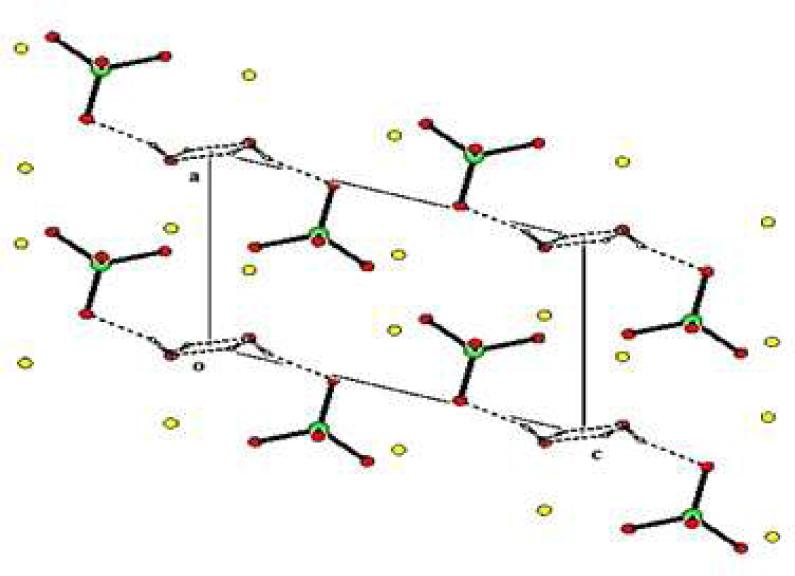The packing arrangement of molecules viewed down the b-axis.
Numerical calculations

From single crystal X-ray diffraction analysis the calculated lattice parameters are: a = 5.4512 Å, b = 4.8726 Å, c = 8.1724 Å, β = 107.291°, showing that the grown crystal belongs to monoclinic system with the space group P21, which agrees with that of reported values .

The valence electron plasma energy, ħωp is calculated using the relation: $ℏωp = 28.8 ZρM$ $$\hslash {\omega _p}{\text{ = 28}}{\text{.8 }}\left[ {\frac{{Z\rho }}{M}} \right]$$

where Z = (2 × ZLi) + (2 × ZH) + (1 × ZS) + (5 × ZO) is the total number of valence electrons, ρ is the density and M is the molecular weight of LSMH.

Penn gap Ep and the Fermi energy EF is, respectively, given by the equations: $Ep= ℏωpε∞−11/2$ $${E_p} \,=\, {\text{ }}\frac{{\hslash{\,\omega\, _p}}}{{{{\left( {{\varepsilon _\infty } \,-\, 1} \right)}^{1/2}}}}$$

$EF = 0.2948ℏωp4/3$ $${E_F}{\text{ = 0}}{\text{.2948}}{\left( {\hslash\,{\omega _p}} \right)^{4/3}}$$

where align is the value of dielectric constant at high frequency (1 MHz in the present case).

The electronic polarizability, α can be obtained using the relation : $α=ℏωp2SOℏωp2SO+3Ep2×MP×0.396×10−24cm−3$ $$\alpha {\text{ = }}\left[ {\frac{{{{\left( \hslash {\omega _p} \right)}^2}S_O}}{{{{\left( {\hslash {\omega _p}} \right)}^2}S_{O} \rm + \rm {3}\it E_p^\rm 2}}} \right]{\text{ }} \times \frac{{M}}{{{P}}}{\text{ }} \times {\text{ 0}}{\text{.396 }} \times {\text{1}}{{\text{0}}^{ - {\text{24}}}}{\text{c}}{{\text{m}}^{ - {\text{3}}}}$$

where S0 is a constant for a particular material which is given by: $S0 = 1−Ep4EF + 13Ep4EF2$ $$S_0{\text{ = 1}} - \left[ {\frac{{{E_p}}}{{4{E_F}}}} \right]{\text{ }} + {\text{ }}\frac{1}{3}{\left[ {\frac{{{E_p}}}{{4{E_F}}}} \right]^2}$$

The value of α obtained from the previous equation agrees with the value obtained by using Clausius-Mossotti equation: $α = 3M4πNaρ×ε∞−1ε∞+2$ $$\alpha {\text{ = }}\frac{{3M}}{{4\pi { N_a}\rho }} \times \frac{{{\varepsilon _\infty } - 1}}{{{\varepsilon _\infty } + 2}}$$

The calculated parameters for the grown crystal are shown in the Table 4 and the values obtained are of the same order as reported for such type of material .

Calculated data for LSMH single crystal.

ParametersValues
Plasma energy [eV]18.86
Penn gap [eV]8.0
Fermi energy [eV]14.80
Polarizability [cm−3] using Penn analysis1.6 × 10−23
Polarizability [cm−3] using Clausius-Mossoti equation1.68 × 10−23
Morphological study

The molecular and crystalline modeling software based on molecular mechanics have been used to build new methods for prediction of crystal morphology. Bravais-Friedel-Donnay-Harker (BFDH) is one of the methods to predict the crystal morphology. From BFDH method a series of possible growth faces (hkl) can be determined from the crystal lattice and symmetry. The crystal morphology of LSMH crystal was simulated by Materials Studio 8.0 program  using BFDH model . The simulated morphology was correlated with the as grown morphology of the crystal shown in Fig. 3. It was found that the simulated morphology predicted by BFDH model matches with the morphology of as grown single crystal of LSMH.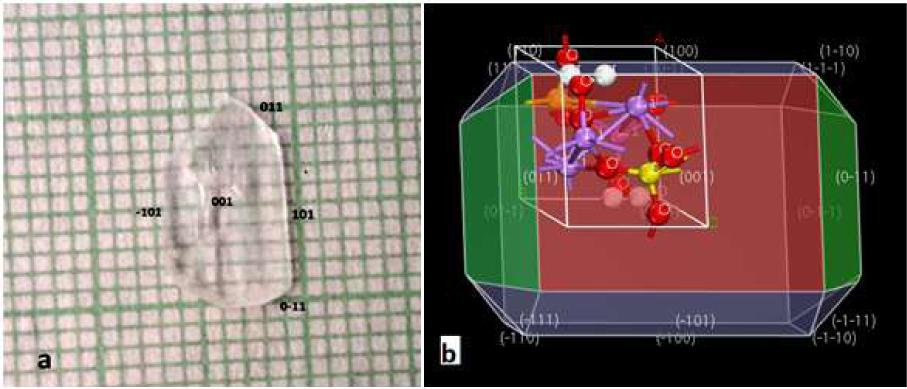Morphology of LSMH crystal (a) as grown and (b) predicted by BFDH model.
Optical studies
Optical transmittance and absorption studies

The optical transmission spectrum of LSMH single crystal is shown in Fig. 4. It is observed from the spectrum that the crystal shows good transmission in the entire visible region and it has been reported that such kind of material finds its application in second harmonic generation devices .The variation of optical absorption coefficient with the photon energy provides information about the band structure and the type of electronic transitions .

The optical absorption coefficient (α) was calculated from the transmittance using the following equation: $α = 2.303log1Td$ $$\alpha {\text{ = }}\frac{{2.303\log \left( {\frac{1}{T}} \right)}}{d}$$

where T is the transmittance and d is the thickness of the crystal. The crystal under study has an optical absorption coefficient (α) obeying the following equation (Tauc’s relation) for high photoenergies (hν): $α = A(hν−Eg)hν$ $$\alpha {\text{ = }}\frac{{A\sqrt {(h\nu - {E_g})} }}{{h\nu}}$$

where A is a constant and Eg is optical band gap of the crystal. Fig. 5 shows the variation of (αhν)2 versus photon energy E(eV), where the energy gap Eg is evaluated by the extrapolation of the linear part . The band gap is found to be 4.49 eV. As a consequence of the wide band gap, the grown crystal has large transmittance in the visible region .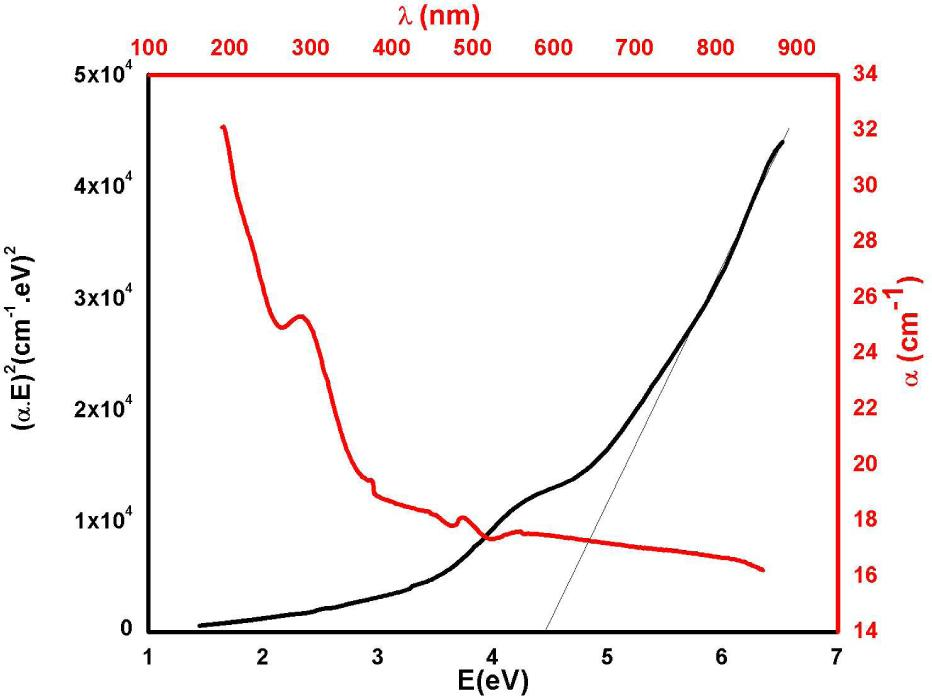Tauc’s plot and variation of absorption coefficient with wavelength for LSMH crystal.

Fig. 5 shows the variation of absorption coefficient (α) with wavelength of LSMH crystal. From the spectrum, a low absorption in the UV and visible region reveals that it is a material possessing NLO properties. The UV cutoff wavelength was found to be 290 nm for the grown crystal. The large band gap and transparency shown by the crystals make them a possible choice for optoelectronic application [39, 46]. The transmittance (T) is given by the relation : $T = (1−R)2exp⁡(−αd)1−R2exp⁡(−2αd)$ $$T{\text{ = }}\frac{{{{(1 - R)}^2}\exp ( - \alpha d)}}{{1 - {R^2}\exp ( - 2\alpha d)}}$$

The reflectance in terms of the optical absorption coefficient can be derived from the equation: $R=1±1−exp⁡(−αd)+exp⁡(αd)1+exp⁡(−αd)$ $${{R = }}\frac{{1 \pm \sqrt {1 - \exp ( - \alpha d) + \exp (\alpha d)} }}{{1 + \exp ( - \alpha d)}}$$

FT-IR analysis

The FT-IR spectrum of LSMH is shown in Fig. 6 and from the spectrum it is evident that the presence of various bands corresponding to different wave numbers confirms that the grown crystal is LSMH. Also the band at 3471 cm−1 is attributed to symmetric stretching of O–H coordinated water molecule, band at 1614 cm−1 is due to H–O–H bending and S–O stretching of $SO42−$$\text{SO}_4^{2-}$ moiety of Li2SO4. The S–O stretching is also confirmed by a band at 1095 cm−1.

The force constant (k) for all these frequency assignments can be determined by using the formula: $k=4π2ω¯e2μ$ $${{k = 4}}{\pi ^2}\bar \omega_e^2\mu$$

where μ is the reduced $mass=MaMbMa+Mbandω¯e$ $\text {mass} {{ }} = {{ }}\frac{{{\rm M_a}{\rm M_b}}}{{{\rm M_a} + {\rm M_b}}}\,\,\,\rm and\,\,\,{\bar \omega _e}$ is the fundamental vibrational frequency. The force constant for fundamental vibrations of the grown crystal are presented in Table 2. The purpose of this calculation is to find the stretching and interaction force constants corresponding to its position in the molecule.

Dielectric characteristics
Variation of dielectric constant and dielectric loss with temperature

Fig. 7 shows variation of dielectric constant (∊′) of LSMH crystal with temperature at different frequencies. It is observed that ∊′ shows almost no variation with temperature up to 105 °C and above this temperature ∊′ changes rapidly showing a peak at around 130 °C. The inset of Fig. 7 shows variation of dielectric constant with temperature at 100 kHz. The possible reason for this peak is either due to structural phase transition or due to dehydration, i.e. removal of water molecule from the LSMH crystal. From literature study we have found that LSMH is non ferroelectric , so the possibility of structural phase transition can be ruled out. The dielectric anomaly is thus due to dehydration of the crystal. As water is a polarized molecule, below 105 °C the water molecule is coordinated (interaction) to different atoms of the crystal which prevents the reorientation of the molecular dipole. With the increase in temperature above 105 °C this interaction breaks down, water molecule orients easily which leads to an increase in dielectric constant that attains the maximum value at 130 °C. The orientational polarization shown by the water molecule, because of its asymmetrical bonding structure results in its permanent dipole moment. Beyond 130 °C a decrease in orientational polarization due to gradual loss of water molecules and irregular motion of permanent dipole moments leads to a decrease in dielectric constant . As such, it is proposed that the anomalous dielectric behavior shown by LSMH crystal is due to the presence of coordinated water molecule which is also supported by TGA data shown in Fig. 16. Thus, the absorption of water molecules by a crystal surface can affect the dielectric constant of the crystal and contribute to dielectric peak as reported in the literature . Beyond 145 °C, the title crystal shows normal behavior and dielectric constant increases with temperature as observed in various polar materials .

Force constant (k) values for different vibrations of LSMH crystals.

Frequency [cm−1]Vibrational assignmentForce constant k [N/m]
3471O–H stretching643
1614S–O stretching1575
1095S–O stretching725

Fig. 8 shows the variation of dielectric loss with temperature at different frequencies and also shows peak at around 130 °C. Since energy is needed for the reorientation of a molecule in order to overcome the surrounding molecules resistance, the orientation process depends on temperature. In the temperature range of 105 °C < T < 130 °C, the increase in dielectric constant occurs because more energy is dissipated for reorienting the permanent dipoles in the direction of applied field which results in an increase in dissipation loss in this temperature range. Thus, it is evident that the peak in dielectric loss is mostly independent of frequency and is a feature of polar dielectrics, where losses due to electrical conduction also occur apart from dipole losses .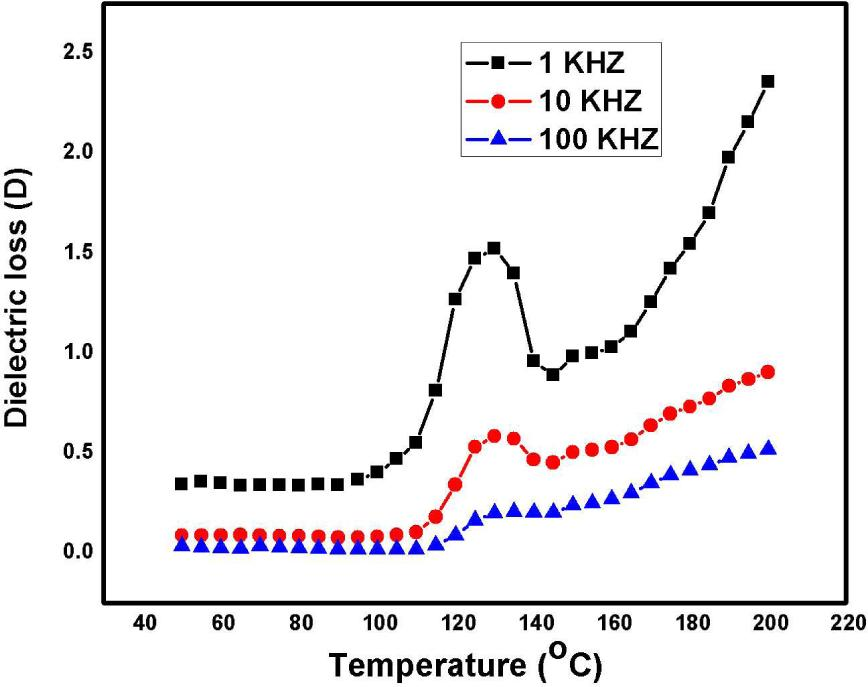Variation of dielectric loss (D) versus temperature for LSMH crystal.
Variation of dielectric constant and dielectric loss with frequency

Fig. 9 shows variation of dielectric constant (∊′) with frequency at different temperatures which represents normal behavior of dielectric materials. The mechanism behind the variation of (∊′) with frequency is polarization phenomena. At low frequency, all the four polarization mechanisms, namely space charge, orientational, electronic and ionic contribute to dielectric constant. The high value of dielectric constant at low frequency may be attributed to interfacial polarization in which the mobile charge carriers are hampered by a physical barrier which stops generation of localized polarization in the crystal. At higher frequencies, the lower value of dielectric constant is due to the gradual loss of significance of these polarizations and material response to the field is missing.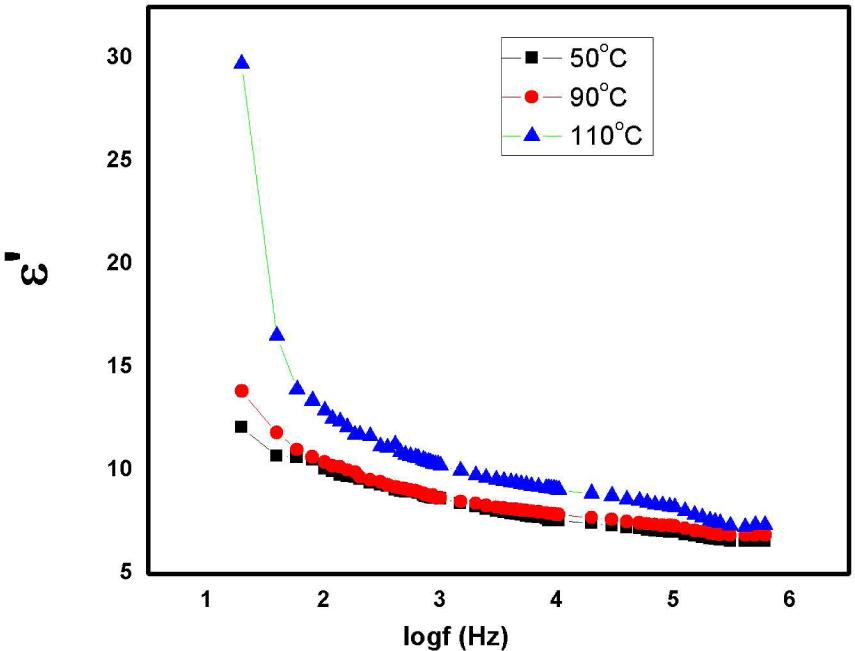Variation of real part of dielectric constant (∊′) versus logf (Hz) for LSMH crystal.

The frequency dependent dielectric loss (D) at different temperatures displayed in Fig. 10 shows normal behavior consisting in that both dielectric constant and dielectric loss show inverse frequency dependence at low frequencies. It is observed that dielectric loss is frequency independent beyond 100 kHz due to dipole rotation, because at higher frequencies the orientational polarization ceases and energy does not need to be spent to rotate the dipoles. At higher frequencies the dipoles fail to follow the field change and react to such fields feebly causing that the dielectric losses are smaller. It is suggested that LSMH crystal shows low frequency dispersion (LFD) or the quasi-DC process (QDC) behavior because of absence of any loss peak in the dielectric dispersion . The lower values of dielectric loss with higher frequencies for this crystal suggest that the material possesses enhanced optical quality with lesser defects and this parameter is of vital importance for nonlinear optical materials and their application .

The real and imaginary parts of susceptibility increase rapidly towards lower frequencies and LSMH crystal seem to obey the power law of the type: χω(n−1) such that 0 < n < 1, where χ is the electric susceptibility of the material which is related to the real part of dielectric constant as χ = ∊′–1. In order to obtain the value of n we have plotted logχ versus logω as shown in Fig. 11 and from the slope, the value of n = 0.9 was obtained. The universal fractional power law is obeyed by the variety of solid materials, including low loss dielectrics, dipolar materials, hopping electronic systems, ionic conductors, semiconductors, p-n junctions, interfacial phenomena and mechanical relaxation .

Electrical conductivity studies
Variation of AC conductivity with temperature

From Fig. 12 it is observed that the increase in electrical conductivity with temperature is due to the motion of charge carriers (polarons and free ions) and AC conductivity shows temperature and frequency dependence. The inset of Fig. 12 shows the variation of AC conductivity with temperature at 1 MHz. The increase in mobility of charge carriers in polymeric and semiconductor materials results in variation of conductivity with temperature and frequency . In LSMH crystal it is observed that the increase in conductivity with temperature from 105 °C to 130 °C is due to dissociation of water molecule into H+ and OH ions which in turn increase the concentration of charge carriers. Such type of conduction is described as protonic conduction . Beyond 130 °C, because of dehydration of water molecule, the conductivity decreases due to the decrease in the concentration of H+ and OH ions as is also observed in the thermal analysis of the crystal (Fig. 16). Since LSMH crystal contains only one water molecule, so the increase in conductivity is not as large as reported for the loss of seven water molecules in crystals . Above 145 °C, after the complete dehydration of LSMH, the increase in conductivity is due to the movement of Li+ ions. So, the conductivity variation is due to both types of ions, that is protons and lithium cations. Thus, the crystal can be used as an electrolyte in solid state batteries at high temperatures .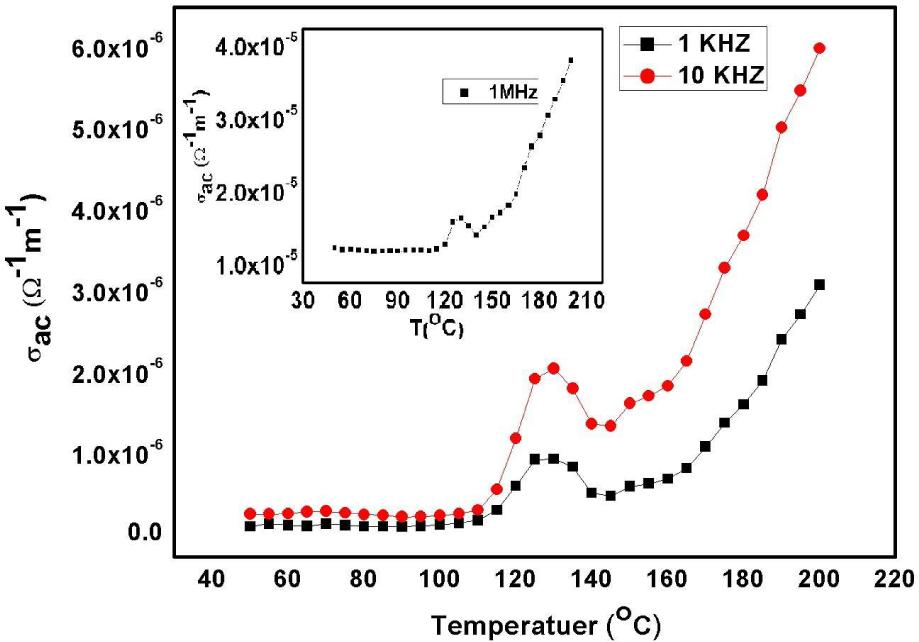Variation of AC conductivity versus temperature for LSMH crystal.

Arrhenius equation σac = σoexp (–Ea/kT) was used to calculate the activation energy of LSMH crystal before the transition temperature (130 °C). Fig. 13 shows the plot of lnσac versus 1000/T at 1 kHz before transition temperature. The activation energy obtained from the slope is 0.24 eV for the crystal.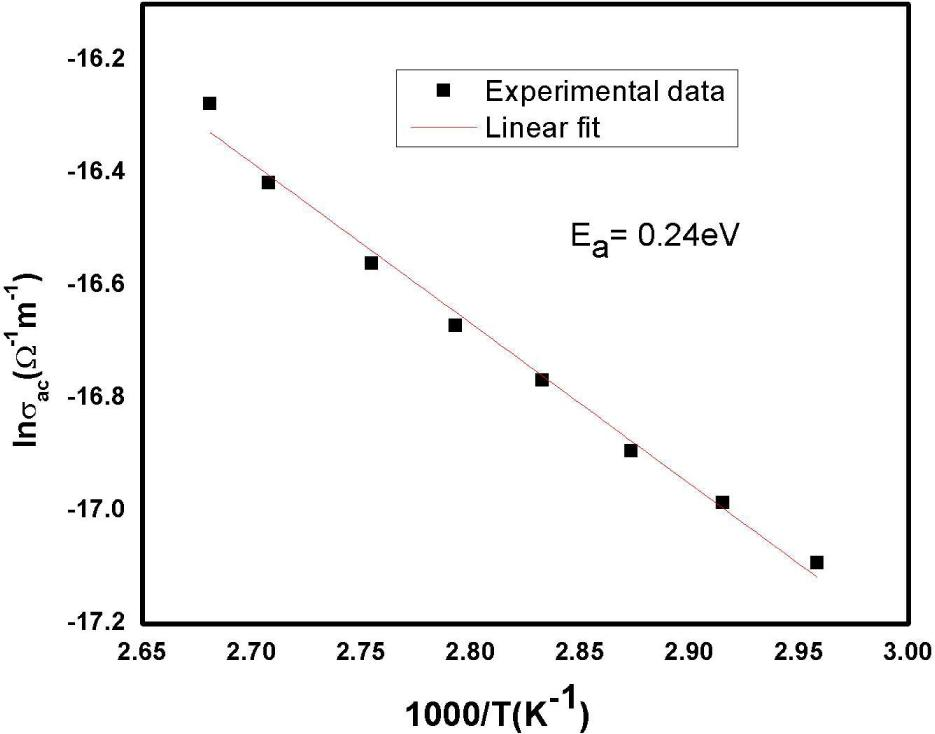Plot of ln σac versus 1000/T at 1 kHz for LSMH crystal.
Variation of AC conductivity with frequency

Earlier, Jonscher showed the variation of electrical conductivity σac with frequency for various solids, including polymers, glasses and crystals . Generally, in conduction band AC conductivity decreases with an increase in frequency while it increases with frequency in case of hopping conduction. Also below phonon frequencies (low frequencies) the ordered solids show no frequency dependence of their conductivity.

In limited frequency region, as shown in Fig. 14, the AC conductivity of the LSMH crystal as a function of frequency seems to obey Jonscher’s universal power law [59, 60]; σ(ω) = σo + Aωs, where σo is the DC conductivity which forms the frequency-independent plateau in the low frequency region, A is temperature dependent constant, and s is temperature dependent power law exponent with values of 0 < s < 1. When s = 0, electrical conduction is frequency independent (DC conduction) that is conduction at very low frequency, and when s > 0, the conduction is frequency dependent (AC conduction) .

In the present study, the value of s is calculated from the plot of ln (σac) versus ln (ω) at 50°C as shown in Fig. 15. The value of s of 0.66, calculated from the slope of this graph confirms that s lies in the range of 0 < s < 1 and obeys power law.This increasing behavior of conductivity with frequency shows hopping conductivity of protons via hydrogen bonds . The proton-proton interaction occurs as a result of electric field which produces directional flow of charge carriers by hopping mechanism. When proton tries to move, it has to pass through the strain field caused by the cloud of virtual thermal phonons thus forming a quasiparticle, like polaron. This quasiparticle disperses at higher frequencies of applied AC field. When the cloud of phonons disperses, protons move and contribute to conductivity .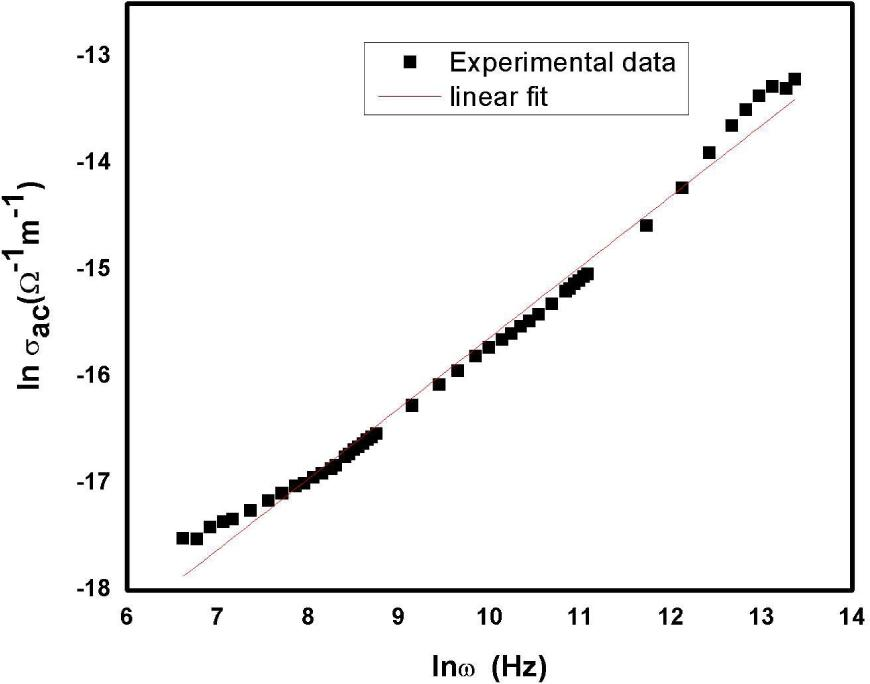Plot of lnσac versus lnω for LSMH crystal at temperature of 50 °C.
Thermal analysis

In order to ascertain the thermal stability of LSMH crystal and correlate the dielectric results with thermal behavior, thermogravimetric analysis was carried out in the nitrogen atmosphere. The TGA/DTG curves are shown in Fig. 16. The TGA curve shows that there is a loss of one water molecule at around 130 °C and the material is stable up to a temperature of about 105 °C. In the first step from 105 °C to 145 °C, the material undergoes a weight loss of about 13.6 %. The weight loss calculated for the proposed formula Li2SO4H2O, when considered for the removal of water molecules is 14.06 %, which is in agreement with the experimental data. The peak in the DTG curve at 130 °C corresponds to a loss of this water molecule. Due to the loss of water, transition in the dielectric behavior is also observed (Fig. 7). The weight loss in the range of 145 °C to 700 °C is only about 1 % which indicates high thermal stability of the title compound .

Microhardness studies

The mechanical strength of LSMH crystal was determined by Vickers microhardness measurement at room temperature. A polished crystal was placed on a platform of a Vickers micro hardness tester, and loads of different magnitudes from 20 g to 100 g were applied over a fixed duration of 5 s. For all these loads, the indentation time was maintained at 5 s. Several indentations were made on the crystal surface with sufficient space for each load, and the diagonal length (d) of the indented impressions was measured. The Vickers hardness number of the materials, HV was determined by the relation: $Hv=1.8544Pd2kg/mm2$ $$H_v\,\,=\,\,\frac{{1.8544P}}{{{d^2}}}\text {kg}/\text {mm}^2$$

where d is the diagonal length of the indentation impression in mm and P is the applied load in kg. The hardness number was found to increase as the load increased, and above 100 g, significant cracks and inclusions were observed, which may be due to the release of internal stress, generated locally by the indentation .

A plot of corresponding hardness values of LFMH versus load is shown in Fig. 17. The work-hardening coefficient (n) of the material is related to the load (p) by the relation: $P=Adn$ $$P\,\, =\, \, A{{{d}}^n}$$

where A is an arbitrary constant. The work-hardening coefficient n of the sample was determined from the slope of the plot of logp versus logd shown in Fig. 18, and it was found to be 1.87, indicating that the crystal belongs to the soft material category. Onitsch  and Hanneman  noted that a value of n between 1.0 and 1.6 indicates a moderately hard material and that a value of n greater than 1.6 represents a soft material. The obtained value of n indicates that the LSMH crystal has a high mechanical strength and can be used in device applications.

Conclusions

Single crystal XRD study showed that LSMH crystallizes into monoclinic structure with a space group P21. Resolving the structure and refinement to final R-value of 0.0174 confirmed the formation of layered structure, mainly due to H bonding. Theoretical parameters, plasma energy, Penn gap, Fermi energy and electronic polarizability have also been calculated for the present system. Polarizability calculated by using Clausius-Mossotti equation and that obtained by using Penn analysis were found to be in agreement. The optical study revealed that the material has a wide optical band gap of 4.49 eV and shows good transmittance in UV-Vis region which makes it suitable as a candidate for optoelectronic application. The FT-IR spectrum confirmed the presence of various functional groups in the grown crystal. Dielectric constant of LSMH crystal obtained from the dielectric study, showed strong dependence on frequency and temperature. The dielectric anomaly observed in the title compound is due to dehydration of crystal. The AC conductivity study showed that the crystal obeys Jonscher’s power law. The conductivity of LSMH in the temperature range up to 200 °C was attributed to the migration of both types of ions, protons and lithium ions. The Vickers hardness test confirmed that the crystal belongs to soft materials.

#### Crystallographic and experimental data.

 Crystal description white block Crystal size 0.5 mm3 × 0.3 mm3 × 0.2 mm3 Empirical formula H2Li2O5S Formula weight 127.96 g Radiation, wavelength MoKα, 0.71073 Å Unit cell dimensions a = 5.4512(8) Å, b = 4.8726(6) Å, c = 8.1724(10) Å, α = 90°, β = 107.291° (3), = 90° Crystal system monoclinic Space group P 21 Unit cell volume 207.26(5) Å−3 No. of molecules per unit cell, Z 2 Temperature 296(2) K Absorption coefficient 0.676 mm−1 F(0 0 0) 128 Scan mode ω scan θ range for entire data collection 2.61° < θ < 28.32° Range of indices h = −6 to 5, k = −6 to 6.1 = −10 to 10 Reflections collected/unique 1348/453 Reflections observed (I > 2σ (I)) 451 Rint 0.0206 Rsigma 0.0193 Structure determination Direct methods Refinement Full-matrix least-squares on F2 No. of parameters refined 82 Final R 0.0174 wR(F2) 0.0472 Weight 1/[σ2(Fo2)+(0.0286P)2+0.0218P]whereP=[Fo2+2Fc2]/3$1/[\sigma^2( {\text F_\text o^2} ) + (0.0286\text P)^2 + 0.0218\text P]\,\,\,\text {where} \,\,\,\text P = [ {\text F_\text o^2} + 2 {\text F_\text c^2} ]/3$ Goodness-of-fit 1.287 Final residual electron density −0.308 eÅ−3 < Δρ < 0.161 eÅ−3

#### Force constant (k) values for different vibrations of LSMH crystals.

Frequency [cm−1]Vibrational assignmentForce constant k [N/m]
3471O–H stretching643
1614S–O stretching1575
1095S–O stretching725

#### Bond lengths [Å], bond angles [°] and torsion angles for LSMH atoms (e.s.d.’s are given in parentheses).

 Bond distances S1–O1 1.4774(16) S1–O2 1.4734(16) S1–O3 1.4598(16) S1–O4 1.475(2) O1–Li1 1.950(4) O2–Li2 1.957(5) Li1–05ix 1.905(4) O5–H2 0.89(6) O5–H1 0.64(5) Bond angles O4–S1–O2 108.88(10) O2–S1–O1 108.88(10) O3–S1–O2 110.14(10) O3–S1–O4 110.56(10) O4–S1–O1 108.89(10) O3–S1–O1 109.46(10) S1–O2–Li2 120.33(14) S1–O1–Li1 119.02(14) O5ix–Li1–O1 107.1(2) Li1–O5–H1 133(5) Li1–O5–H2 106(3) H2–O5–H1 115(6) Torsion angles O3–S1–O2–Li2 –41.44(18) O4–S1–O2–Li2 –162.82(15) O4–S1–O1–Li1ii 48.0(2) O3–S1–O1–Li1ii –73.0(2) S1–O1–Li1–O5ix 95.49(19)

#### Intermolecular hydrogen bonding of the O–H…O type.

[Å][Å][Å][°]
O5–H1…O3i0 652.302.90155
O5–H2… O5ii0.892.112.96161

#### Calculated data for LSMH single crystal.

ParametersValues
Plasma energy [eV]18.86
Penn gap [eV]8.0
Fermi energy [eV]14.80
Polarizability [cm−3] using Penn analysis1.6 × 10−23
Polarizability [cm−3] using Clausius-Mossoti equation1.68 × 10−23

Bosshard C., Sutter K., Schlesser R., Gunter P., J. Opt. Soc. Am. B, 10 (1993), 869.BosshardC.SutterK.SchlesserR.GunterP.J. Opt. Soc. Am. B10199386910.1364/JOSAA.10.000869Search in Google Scholar

Xu D., Jiang M., Tan Z., Acta Chim. Sinica, 41 (1983), 570.XuD.JiangM.TanZ.Acta Chim. Sinica411983570Search in Google Scholar

Calark R.S., Photonic Spectra, 22 (1988), 135CalarkR.S.Photonic Spectra22198813510.2307/2546588Search in Google Scholar

Prasad P.N., Williams D.J., Introduction to Nonlinear Optical Effects in Molecules and Polymers, Wiley, New York, 1991.PrasadP.N.WilliamsD.J.Introduction to Nonlinear Optical Effects in Molecules and PolymersWileyNew York1991Search in Google Scholar

Robert R., Raj C.J., Krishnan S., Uthrakumar R., Dinakaran S., Das S.J., Physica B, 405 (2010), 3248.RobertR.RajC.J.KrishnanS.UthrakumarR.DinakaranS.DasS.J.Physica B4052010324810.1016/j.physb.2010.04.053Search in Google Scholar

Uthrakumar R., Vesta C., Raj C.J., S. Dinakaran, R. Christhudhas R., Das S.J., Cryst. Res. Technol., 43 (2008), 28.UthrakumarR.VestaC.RajC.J.DinakaranR.ChristhudhasR.DasS.J.Cryst. Res. Technol.4320082810.1002/crat.200710986Search in Google Scholar

Zeiler G.E., Z. Kristallogr., 89 (1934), 456.ZeilerG.E.Z. Kristallogr.89193445610.1524/zkri.1934.89.1.456Search in Google Scholar

Larson A.C., Helmhotz L., J. Chem. Phys., 22 (1954), 2049.LarsonA.C.HelmhotzL.J. Chem. Phys.221954204910.1063/1.1739990Search in Google Scholar

Ozerov R.P., Fykin L.E., N.V. Rannev N.V., Zhdanov G.S., Doklady Akademii Nauk SSSR, 148 (1963), 1069.OzerovR.P.FykinL.E.N.V. RannevN.V.ZhdanovG.S.Doklady Akademii Nauk SSSR14819631069Search in Google Scholar

Becker P., Ahrweiler S., Held P., Schneeberger H., Bohaty L., Cryst. Res. Technol., 38 (2003), 881.BeckerP.AhrweilerS.HeldP.SchneebergerH.BohatyL.Cryst. Res. Technol.38200388110.1002/crat.200310107Search in Google Scholar

Boopathi K., Rajesh P., Ramasamy P., J. Cryst. Growth, 345 (2012), 1.BoopathiK.RajeshP.RamasamyP.J. Cryst. Growth3452012110.1016/j.jcrysgro.2012.01.036Search in Google Scholar

Lang S.B., Phys. Rev. B, 4 (1971), 3603.LangS.B.Phys. Rev. B41971360310.1103/PhysRevB.4.3603Search in Google Scholar

Bayarjargal L., Bohaty L., Acta Crystallogr. A, 61 (2005), 393.BayarjargalL.BohatyL.Acta Crystallogr. A61200539310.1107/S0108767305083339Search in Google Scholar

Lundgren J.O., Kvick A., Karppinen R., Liminga R., Abrahams S.C., J. Chem. Phys., 80 (1984), 423.LundgrenJ.O.KvickA.KarppinenR.LimingaR.AbrahamsS.C.J. Chem. Phys.80198442310.1063/1.446465Search in Google Scholar

Bayarjargal L., Cryst. Res. Technol., 43 (2008), 1138.BayarjargalL.Cryst. Res. Technol.432008113810.1002/crat.200800311Search in Google Scholar

Canterford R.P., Nino F., J. Phys. Chem. C, 8 (1975), 1750.CanterfordR.P.NinoF.J. Phys. Chem. C81975175010.1088/0022-3719/8/11/028Search in Google Scholar

Priya R., Krishnan S., Raj C.J., Das S.J., Cryst. Res. Technol., 44 (2009), 1272.PriyaR.KrishnanS.RajC.J.DasS.J.Cryst. Res. Technol.442009127210.1002/crat.200900504Search in Google Scholar

Czapla Z., Debska M., Lebedeva E., Pirozerski A., Acta Phys. Pol. A, 99 (2001), 539.CzaplaZ.DebskaM.LebedevaE.PirozerskiA.Acta Phys. Pol. A99200153910.12693/APhysPolA.99.539Search in Google Scholar

Ueda K., Kaminskii A.A., Laser Phys., 15 (2005), 1509.UedaK.KaminskiiA.A.Laser Phys.1520051509Search in Google Scholar

Gavrilova N.D., Maksimov E.G., Noviko V.K., Drozhdin S.N., Fizika Tverdogo Tela, 27 (1985), 2597.GavrilovaN.D.MaksimovE.G.NovikoV.K.DrozhdinS.N.Fizika Tverdogo Tela2719852597Search in Google Scholar

Mageshwari P.S.L., Priya R., Krishnan S., Joseph V., Das S.J., Optik, 125 (2014), 2289.MageshwariP.S.L.PriyaR.KrishnanS.JosephV.DasS.J.Optik1252014228910.1016/j.ijleo.2013.10.121Search in Google Scholar

Kawada K., Mcghil A.R., Labes M.M., J. Chem. Phys., 52 (1970), 3121.KawadaK.McghilA.R.LabesM.M.J. Chem. Phys.521970312110.1063/1.1673447Search in Google Scholar

Hali M., Boysen D.A., Chisholom C.R., Meri R.B., Nature, 410 (2001), 910.HaliM.BoysenD.A.ChisholomC.R.MeriR.B.Nature410200191010.1038/35073536Search in Google Scholar

Lim A.R., J. Phys.-Condens., Mat., 19 (2007), 11621.LimA.R.J. Phys.-Condens., Mat.19200711621Search in Google Scholar

Heed B., Lunden A., Schroeder K., Electrochim. Acta, 22 (1977), 705.HeedB.LundenA.SchroederK.Electrochim. Acta22197770510.1016/0013-4686(77)80022-4Search in Google Scholar

Deshpande V.K., Singh K., Solid State Ionics, 6 (1982), 151.DeshpandeV.K.SinghK.Solid State Ionics6198215110.1016/0167-2738(82)90081-9Search in Google Scholar

Goodenough J.B., P. Roy. Soc. A-Math. Phy., 393 (1984), 215.GoodenoughJ.B.P. Roy. Soc. A-Math. Phy.393198421510.1098/rspa.1984.0055Search in Google Scholar

Sheldrick G.M., Acta Crystallogr. A, 64 (2008), 112.SheldrickG.M.Acta Crystallogr. A64200811210.1107/S0108767307043930Search in Google Scholar

Farrugia L.J., J. Appl. Crystallogr., 30 (1997), 565.FarrugiaL.J.J. Appl. Crystallogr.30199756510.1107/S0021889897003117Search in Google Scholar

Farrugia L.J., J. Appl. Crystallogr., 32 (1999), 837.FarrugiaL.J.J. Appl. Crystallogr.32199983710.1107/S0021889899006020Search in Google Scholar

Nardelli M., J. Appl. Crystallogr., 28 (1995), 659.NardelliM.J. Appl. Crystallogr.28199565910.1107/S0021889895007138Search in Google Scholar

Spek A.L., Acta Crystallogr. D, 65 (2009), 148.SpekA.L.Acta Crystallogr. D65200914810.1107/S090744490804362XSearch in Google Scholar

Larson A.C., Acta Crystallogr., 18 (1965), 717.LarsonA.C.Acta Crystallogr.18196571710.1107/S0365110X65001652Search in Google Scholar

Ravindra N.M., Bharadwaj R.P., K.S. Kumar K.S., Srivastava V.K., Infrared Phys. Techn., 21 (1981), 369.RavindraN.M.BharadwajR.P.K.S. KumarK.S.SrivastavaV.K.Infrared Phys. Techn.21198136910.1016/0020-0891(81)90045-2Search in Google Scholar

Ravindra N.M., Srivastava V.K., Infrared Phys. Techn., 67 (1980), 20.RavindraN.M.SrivastavaV.K.Infrared Phys. Techn.6719802010.1016/0020-0891(80)90009-3Search in Google Scholar

Krishnan S., Raj C.J., Robert R., Ramanand A., DAS S.J., Solid State Electron., 52 (2008), 1157.KrishnanS.RajC.J.RobertR.RamanandA.DasS.J.Solid State Electron.522008115710.1016/j.sse.2008.03.015Search in Google Scholar

Kun Z., Jun L., Jianhong L., Yang J., Chinese J. Chem. Eng., 20 (2012), 602.KunZ.JunL.JianhongL.YangJ.Chinese J. Chem. Eng.20201260210.1016/S1004-9541(11)60224-3Search in Google Scholar

Camacho D.M., Roberts K., Lewtas K., More I., J. Cryst. Growth, 416 (2015), 47.CamachoD.M.RobertsK.LewtasK.MoreI.J. Cryst. Growth41620154710.1016/j.jcrysgro.2015.01.006Search in Google Scholar

Krishnakumar V., Nagalakshmi R., Spectrochim. Acta A, 61 (2005), 499.KrishnakumarV.NagalakshmiR.Spectrochim. Acta A61200549910.1016/j.saa.2004.04.014Search in Google Scholar

John Xavier K.V., Spectrochim. Acta A, 60 (2005), 709.JohnXavierK.V.Spectrochim. Acta A60200570910.1136/thx.2005.043760Search in Google Scholar

Roshan A., Joseph S.C.,Mater. Lett., 49 (2001), 299.RoshanA.JosephS.C.Mater. Lett.49200129910.1016/S0167-577X(00)00388-8Search in Google Scholar

Maheswaran V.V., Sherwood S., Bhat J.N., J. Cryst. Growth, 179 (1997), 605.MaheswaranV.V.SherwoodS.BhatJ.N.J. Cryst. Growth179199760510.1016/S0022-0248(97)00137-1Search in Google Scholar

Ciupinaa T.N., Prodana V., Rusub G., Gheorghies G.I., Vasilec C., J. Optoelectron. Adv. M., 6 (1967), 211.CiupinaaT.N.ProdanaV.RusubG.GheorghiesG.I.VasilecC.J. Optoelectron. Adv. M.61967211Search in Google Scholar

Krishnan S., Raj C.J., Priya S.M., Robert R., Dinakaran S., DAS S.J., Cryst. Res. Technol., 8 (2008), 845.KrishnanS.RajC.J.PriyaS.M.RobertR.DinakaranS.DasS.J.Cryst. Res. Technol.8200884510.1002/crat.200711102Search in Google Scholar

Eya D.O.O., Ekpunobi A.J., Okeke C.E., Acad. Open Internet J., 17 (2006), 1311.EyaD.O.O.EkpunobiA.J.OkekeC.E.Acad. Open Internet J.1720061311Search in Google Scholar

Krishnakumar V., John Xavier R., Spectrochim. Acta A, 60 (2004), 709.KrishnakumarV.John XavierR.Spectrochim. Acta A60200470910.1016/S1386-1425(03)00281-6Search in Google Scholar

Denton R.E., Campbell R.D., Tomlin S.G., J. Phys. D Appl. Phys., 5 (1972), 852.DentonR.E.CampbellR.D.TomlinS.G.J. Phys. D Appl. Phys.5197285210.1088/0022-3727/5/4/329Search in Google Scholar

Shah M.D., Want B., Curr. Appl. Phys., 15 (2015), 64.ShahM.D.WantB.Curr. Appl. Phys.1520156410.1016/j.cap.2014.11.002Search in Google Scholar

Soukiassian A., Tagantsev A., Setter N., Appl. Phys. Lett., 97 (2010), 192903.SoukiassianA.TagantsevA.SetterN.Appl. Phys. Lett.97201019290310.1063/1.3505930Search in Google Scholar

Ahmad M.M., Makhlouf S.A., Khalil K.M.S., J. Appl. Phys., 100 (2006), 094323.AhmadM.M.MakhloufS.A.KhalilK.M.S.J. Appl. Phys.100200609432310.1063/1.2364382Search in Google Scholar

Sjostrom J., Mattsson J., Bergman R., Johansson E., Josefsson K., Svantesson D., Swenson J., Phys. Chem. Chem. Phys., 12 (2010), 10452.SjostromJ.MattssonJ.BergmanR.JohanssonE.JosefssonK.SvantessonD.SwensonJ.Phys. Chem. Chem. Phys.1220101045210.1039/c001275kSearch in Google Scholar

Tareev B., Physics of Dielectric Materials, Mir Publishers, Moscow, 1975.TareevB.Physics of Dielectric MaterialsMir PublishersMoscow1975Search in Google Scholar

Jonscher A.K., Colloid Polym. Sci., 253 (1975), 231.JonscherA.K.Colloid Polym. Sci.253197523110.1007/BF01470233Search in Google Scholar

Balarew C., Dushlew R., J. Solid State Chem., 55 (1984), 1.BalarewC.DushlewR.J. Solid State Chem.551984110.1016/0022-4596(84)90240-8Search in Google Scholar

Jonscher A.K., IEEE T. Dielect. In., 27 (1992), 407.JonscherA.K.IEEE T. Dielect. In.27199240710.1109/14.142701Search in Google Scholar

Dutta P., Biswas S., Ghosh M., De S.K., Chatterjee S., Synthetic Met., 122 (2001), 455.DuttaP.BiswasS.GhoshM.DeS.K.ChatterjeeS.Synthetic Met.122200145510.1016/S0379-6779(00)00588-9Search in Google Scholar

Higuchi T., Tsukamoto T., Yamaguchi S., Sata N., Hiramoto K., Ishigame M., Shin S., J. Appl. Phys., 41 (2002), 6440.HiguchiT.TsukamotoT.YamaguchiS.SataN.HiramotoK.IshigameM.ShinS.J. Appl. Phys.412002644010.1143/JJAP.41.6440Search in Google Scholar

Zhu B., Lai Z.H., Mellander B.E., Solid State Ionics, 70 (1994), 125.ZhuB.LaiZ.H.MellanderB.E.Solid State Ionics70199412510.1016/0167-2738(94)90296-8Search in Google Scholar

Jonscher A.K., Nature, 267 (1977), 673.JonscherA.K.Nature267197767310.1038/267673a0Search in Google Scholar

Jonscher A.K., J. Mater. Sci., 16 (1981), 2037.JonscherA.K.J. Mater. Sci.161981203710.1007/BF00542364Search in Google Scholar

Ye Z.G., Handbook of Dielectric, Piezoelectric and Ferroelectric Materials, Woodhead Publishing Limited, Cambridge, 2008.YeZ.G.Handbook of Dielectric, Piezoelectric and Ferroelectric MaterialsWoodhead Publishing LimitedCambridge200810.1533/9781845694005Search in Google Scholar

Vuilleumier R., Borgis D., Nat. Chem., 4 (2012), 432.VuilleumierR.BorgisD.Nat. Chem.4201243210.1038/nchem.1365Search in Google Scholar

Balakrishnan T., Ramamurthi K., Mater. Lett.,62 (2008), 65.BalakrishnanT.RamamurthiK.Mater. Lett.6220086510.1016/j.matlet.2007.04.072Search in Google Scholar

Onitsch E.M., Mikroskopie, 95 (1956), 12.OnitschE.M.Mikroskopie9519561210.1016/0001-6918(56)90010-5Search in Google Scholar

Hanneman M., Metall. Manch., 23 (1941), 135.HannemanM.Metall. Manch.23194113510.1080/03461238.1941.10406171Search in Google Scholar

Wilsonon A.J.C. (Ed.), International Tables for Crystallography. Volume C: Mathematical, physical and chemical tables, Kluwer Academic Publishers, Dordrecht, 1992.WilsononA.J.C.International Tables for Crystallography. Volume C: Mathematical, physical and chemical tablesKluwer Academic PublishersDordrecht1992Search in Google Scholar

• #### Growth, spectral, optical, dielectric and third order nonlinear studies on 2-amino 4-methylpyridinium salicylate single crystals

Recommended articles from Trend MD